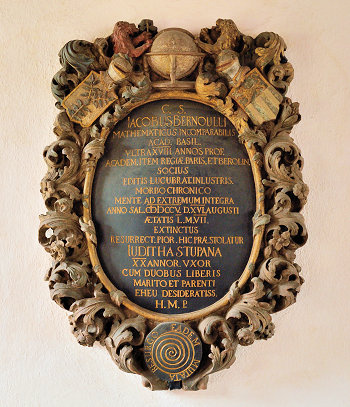Search IntMath
Close

# Lemniscate of Bernoulli

Page by Murray Bourne, IntMath.com. Last updated: 17 December 2019.

## Background

Jacob Bernoulli (1655 - 1755) was a brilliant Swiss mathematician who discovered and developed a broad range of mathematical concepts, including the value of e, differential equations and number theory.

He described what's now called the Lemniscate of Bernoulli in 1694 as a modification of the Ellipse. Both the ellipse and the lemniscate (from the Latin "lēmniscātus" meaning "decorated with ribbons") start with two fixed points, the foci (focuses).

Whereas an ellipse has the property that the sum of the distances from the 2 foci is constant, the Lemniscate has the property that the product of the distances from the foci is constant. You can see this in action in the animation below. The product of the distances remains contant at 0.5.

In the animation, for the distance from the origin to the focus points we've used the value a=sqrt(2)/2~~0.7071, giving us a^2=2/4=0.5.

The first focal point F1 is at (-a,0) ~~ (-0.7071,0), while F2 is the point (a,0)~~ (0.7071,0).

So the distance between the two foci is "F"_1"F"_2 = 2a = sqrt(2).

The point P begins its motion at the point (1,0).

So at the beginning, the distance F1P ≈ 1.7071 and F2P = 1 − 0.7071 ≈ 0.2929, giving us:

F1P × F2P = 1.7071 × 0.2929 = 0.5.

As point P moves, you can see the product of the distance F1P × F2P remains constant at 0.5.

### Relation to a hyperbola

A lemniscate has the neat property that a normal (line at right angles) to the segment OP (where O is the origin and P is a point on the lemniscate) traces out the two arms of a hyperbola.

In this case, the hyperbola you see is x^2 - y^2 = 1.

### Things to do

This is a simple applet. You can choose "show hyperbola" to see the hyperbola mentioned above, and you can pause the motion at any time to see what's going on.

F1P = F2P =
F1P × F2P =

## Formula for Lemniscate of Bernoulli

The general formula, in parametric equations, for the lemniscate is:

x = (acos(t))/(1+sin^2(t))

y= (acos(t)sin(t))/(1+sin^2(t))

0 <= t <= 2pi

## Jacob Bernoulli's Tombstone - Archimedean and Logarithmic SpiralsJacob Bernoulli headstone, showing spiral at the botttom [Basel Catherdral. Photo by Wladyslaw Sojka - Own work, FAL]

One of Jacob Bernoulli's dying wishes was to have a logarithmic spiral on his tombstone, with the motto "Eadem mutata resurgo" (Although changed, I rise again the same).

He felt that such a spiral "may be used as a symbol, either of fortitude and constancy in adversity, or of the human body, which after all its changes, even after death, will be restored to its exact and perfect self."

Either the engravers didn't know what a logarithmic spiral looked like, or for some other reason, in fact the tombstone ended up with an Archimedean spiral.

### Model of the spiral

In the applet below I've modeled the Archimedean spiral using the parametric equations:

x = at sin(πt)
y = −at cos(πt)

The variable t ranges between 0 and , and then a circle of radius completes the pattern.

It was interesting "stepping into the engraver's shoes" for this exercise. You'll notice the spiral doesn't start exactly at the origin (the center of the circular stone slab), and the circle that rounds out the design is also not centered, and not quite at the same place as the spiral's center. He did his best, I guess.

Since Archimedean spirals have equal distances between the arms of the spiral, this would have made it easier to draw the design on the stone.

Length of an Archimedean Spiral where we use calculus to find the length of such a curve.

Curvilinear Motion where we see how parametric equations describe a curve.

### Respecting Bernoulli's wishes

I included the logarithmic spiral (3rd choice) that Bernoulli wanted for his tomb stone. Having done so, I can begin to understand why the engraver was not happy to go with that spiral. Apart from being harder to draw on the original stone, the grooves at the center are quite close to each other and it may have been very difficult to chisel it successfully. (I'm actually starting my curve at t = 3, not t = 0, in order to get reasonable separation between the arms of the curve.)

For the logarithmic spiral, I've used the following parametric equations:

x = aekt cos(πt)
y = aekt sin(πt)